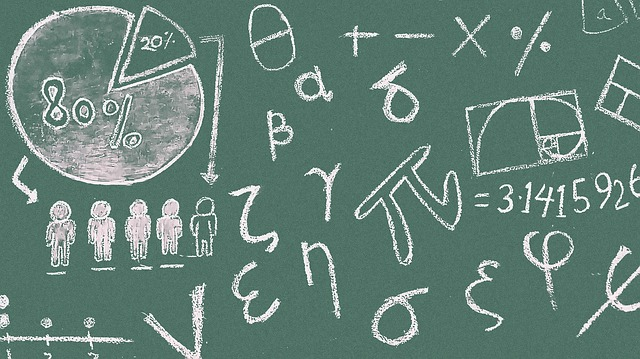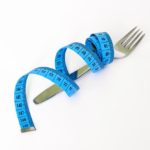in

# Everyday Math Tricks and Tips

We use math every day. Many people think math is scary, but it can be easy when you have the correct process in place. Here are some math tricks to help you out.

As a Math teacher/tutor and home instructor, I have learned from students and colleagues several tricks for Mathematics that I think every child and adult could use. Each makes doing simple maths faster and more efficient and could even surprise a friend with how quickly you reach an answer.

## Nine times tables

Some children find the time’s tables difficult to learn, and having some fluency of them is key to future math abilities when you can’t use or don’t have a calculator. One such way to know your 9 times tables up to 10 is with your hands.

Hold both hands in front of you with your palms facing out.
Consider a times table of nine, say 9 times 4.
Since you are multiplying 9 times 4, put down your 4th finger.
The total number of fingers to the left of the downed finger is the first digit of the answer. In this case, the first digit is 3.
The total number of fingers to the right of the downed finger is the second digit of the answer. In this case, the second digit is 6.
Therefore, 9 times 4 is 36.

## Divisibility Rules

At some point or another, all grade school teachers attempt to teach early elementary students logic in mathematics. In my opinion, this is even harder than teaching the actual early calculations of math. Division is already a hard enough concept to master and understand, but to use logic to determine whether a number can be divided by another is even more difficult. I have found that instead of memorizing up to 7 sentences of divisibility rules, only a handful are necessary and useful and they are much easier to present as “tricks” rather than rules. (Kids hate rules, in case you don’t remember from your days as one)

Trick #1 – The easiest trick. If the number is even, it is divisible by two. Even/odd is a simple concept to grasp. (EX: 18 is even so therefore it is divisible by 2)

Trick #2 – Add the digits of the number up, if the sum is divisible by 3, then the original number is too. This is not too difficult because in most 2 and even 3 digit numbers, the sum is a number much easier to remember if it is divisible by 3. (EX: 18,  1 + 8  is 9 and 9 is divisible by 3, so 18 is too)
If the sum of the digits is still too difficult to know if it can be divided by 3, then apply the rule to that number and try again. If the second sum is divisible by 3 then the first sum is too and then the original number is divisible by 3 as well.

Trick #3 – If the number passes Trick #1 and Trick #2, then the number is divisible by 2 and 3. Which means the number could be divided by the product of 3 and 2, which is 6. (Ex: 18 is divisible by 2 and 3, which means it is also divisible by 6. 18 divided by 6 is 3)

Trick #4 – The toughest trick. If the sum of the digits of the number is divisible by 9, then the whole number is divisible by nine. (Ex: 18. 1 plus 8 is 9. 9 is divisible by 9, therefore 18 is divisible by nine.)
Tricks for divisibility of 4, 5 and 7 are either unnecessary or evolve from knowing the others.

## Greatest Common Factor and Least Common Multiple (GCF and LCM)

Knowing the difference between a factor and multiple is a memorizing MUST in math. A Factor is one part of a pairing of numbers that when multiplied give another number. (EX: 3 x 2 is 6, 3 and 2 are FACTORS of 6. However, so is 1 and 6 because 1 x 6 is also 6) A multiple is one of the infinite number of answers a number has when multiplied by another number. (Ex: 2 can be multiplied by ANY other number, so its MULTIPLES are 2, 4, 6, 8 ….and so on because you can always multiply 2 by the next number) After this is clearly understood by students, then it comes time when they must be able to look at two numbers and determine what is the GREATEST(Largest) factor and the LEAST(smallest) multiple that is the same between the numbers. However, there is one method that will find BOTH answers in one process that doesn’t involve long prime factorization trees or listing endless factors and multiples. I call it, the upside down birthday cake method.
Start by writing both numbers side by side.   (12 and 18)

Then look at your divisibility tricks from earlier and try to find a common trick that works for both. (In this case, 12 and 18 are both even, so Trick #1 is used and both can divide by 2.) Write a 2 to the left of the 12 and 18 and apply the division and re-write the answers of the division below the original two numbers and box the original two in. It should look like the image below.

Repeat the previous step for the two new numbers. (In this case, 6 and 9 are both divisible by 3)

Once the numbers can NOT be divided by the same number anymore, STOP. The GCF and the LCM are easily found in the picture, with some multiplying. The GCF is the numbers on the LEFT side multiplied together. (2 times 3 is 6. The greatest common factor between 12 and 18 is 6) The LCM is ALL of the numbers outside the upside down cake multiplied together. (2 times 3 times 2 times 3, which is 36. The least common multiple of the two numbers is 36.) The LCM is especially easier to remember because if you were to circle all the outside numbers in big bubble, it would make a giant “L” on the paper.

## Math Tricks

There are simple ways to to make math simple. We can use our fingers, think about numbers differently, or use common reference points. Math doesn’t have to be scary if you have the correct tools and a roadmap.

This article was written by Victor Gagliano you can contact him for tutoring.1. Awesome Math Tips for students of any age. I will be sharing this with my kids.

2. Math is scary. Thanks for these tricks I’ll pull this article up when I have to do my taxes.

3. Wow. I’m going to start using math more. Thanks for these helpful tricks and tips!

5. Hello.
If you need another writer for a guest post. I would be happy to write one. Let me know.

6. Great article.

7. Thanks for the ideas you are sharing on this blog site.

8. I’ve always struggled with math. Where was this back when I was in school?! This would have been so helpful!### New App is Facebook of Podcasts